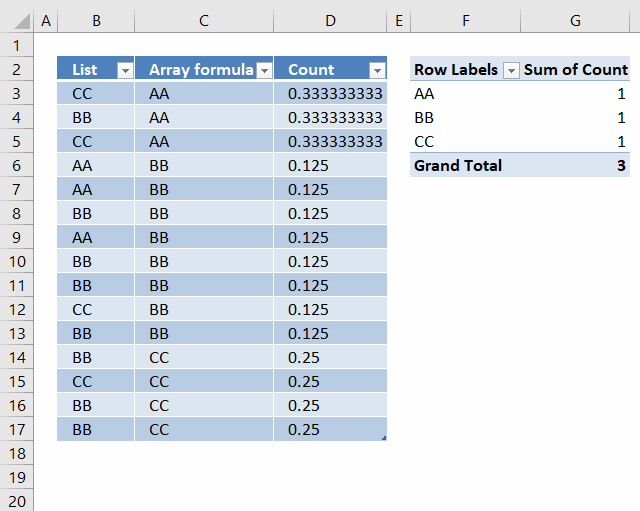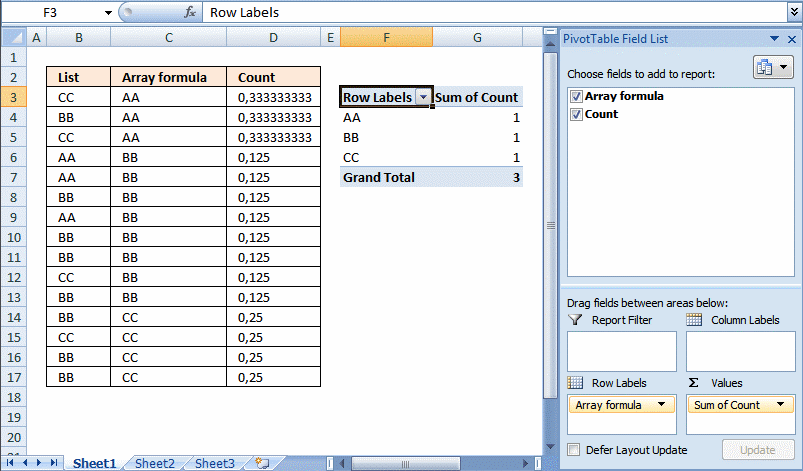Author: Oscar Cronquist Article last updated on January 22, 2019I have a small problem that I am not sure on how to solve.
I now have a list of 15 cells with 3 unique values (arr formula in all 15 cells). When I use this into a pivot table, I want to get the count as 3 since that is the unique count. But I am getting 15 as the value!
I am assuming that the pivot table is reading the formula as a value and counting it. Any way to circumvent that?

Update: It is now possible to count unique distinct values in a Pivot Table. You need Excel 2013 or a later version.

I don´t think it is possible to count unique values in a pivot table unless you add another column to your table or use VBA:  Improve your Excel Pivot Table to count unique values or itemsFormula in D3:

=1/COUNTIF(\$C\$3:\$C\$17, C3)

Copy cell D3 (Ctrl + c). Paste (Ctrl+ v) on cell range D4:D17.

Setup pivot table

1. Press with left mouse button on and drag Array formula to Row Labels
2. Press with left mouse button on and drag Count to Values

### How the formula in cell D3 works

#### Step 1 - Construct the COUNTIF function

COUNTIF(range,criteria) counts the number of cells within a range that meet the given condition.

Select cell D3.

Type =COUNTIF(

Select cell range C3:C17

Press F4. You have now created absolute cell references to cell range C3:C17.
=COUNTIF(\$C\$3:\$C\$17

Now create a relativ cell reference to cell C3.
=COUNTIF(\$C\$3:\$C\$17, C3)

=COUNTIF(\$C\$3:\$C\$17, C3)

becomes

=COUNTIF({"AA"; "AA"; "AA"; "BB"; "BB"; "BB"; "BB"; "BB"; "BB"; "BB"; "BB"; "CC"; "CC"; "CC"; "CC"}, "AA")

and returns 3. There are three AA in the array.

#### Step 2 - Divide 1 with the number of counted values

=1/COUNTIF(\$C\$3:\$C\$17, C3)

becomes

=1/3

and returns

0.333333333333333 in cell D3.

### Get the Excel filecount-unique-values-in-a-pivot-table.xlsx

### Get the Excel filecount-unique-values-in-a-pivot-table-1.xlsx

Recommended blog posts
Excel 2007 pivot table: Count unique distinct records (rows)